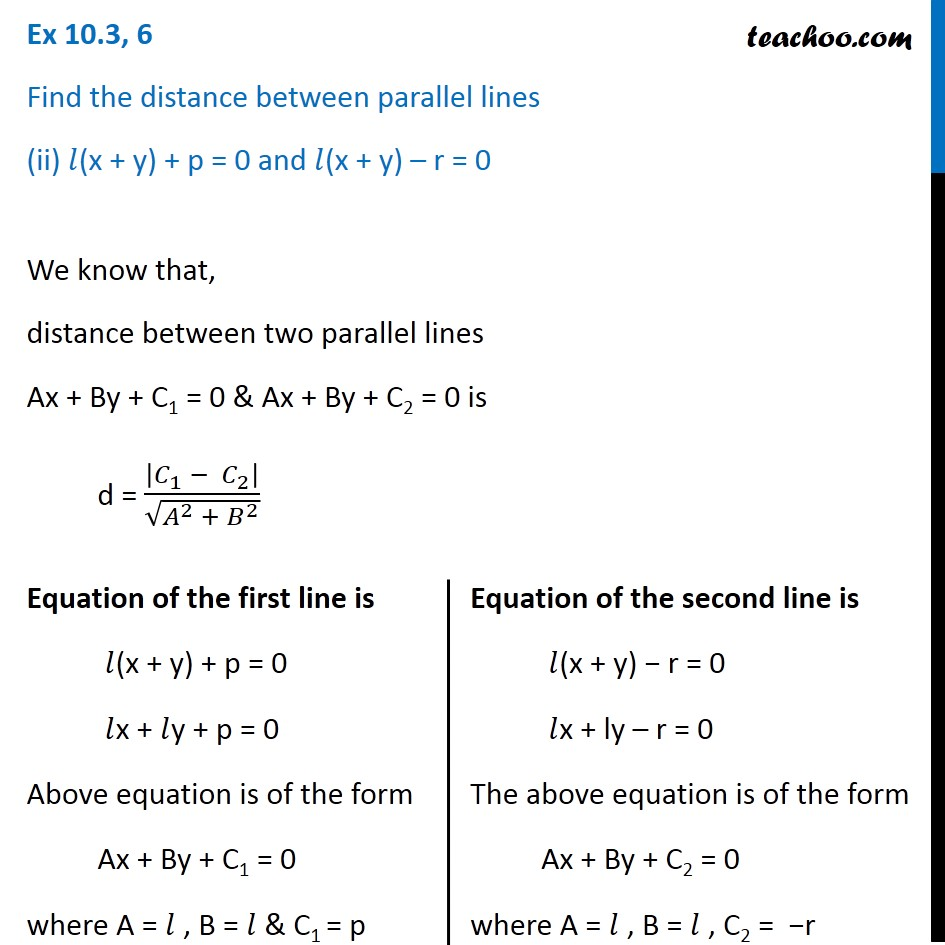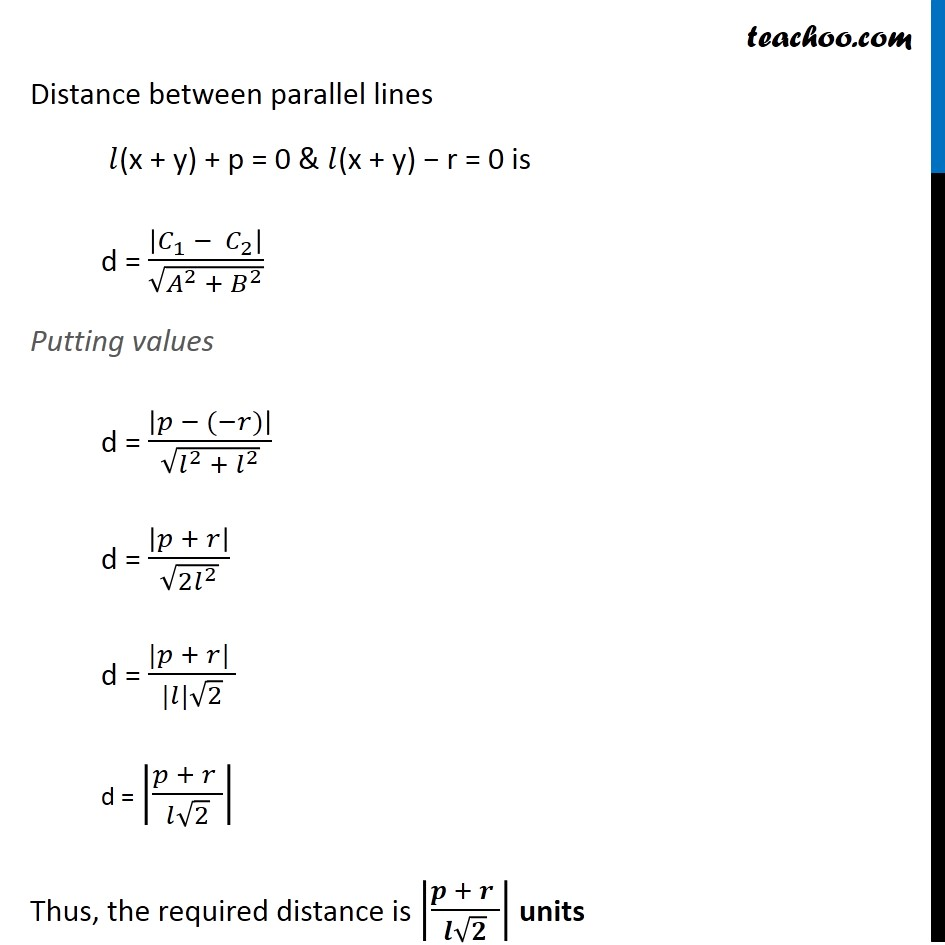Ex 9.3

Chapter 9 Class 11 Straight Lines
Serial order wiseLearn in your speed, with individual attention - Teachoo Maths 1-on-1 Class

### Transcript

Ex 9.3, 6 Find the distance between parallel lines (ii) 𝑙(x + y) + p = 0 and 𝑙(x + y) – r = 0 We know that, distance between two parallel lines Ax + By + C1 = 0 & Ax + By + C2 = 0 is d = |𝐶_1 − 𝐶_2 |/√(𝐴^2 + 𝐵^2 ) Equation of the first line is 𝑙(x + y) + p = 0 𝑙x + 𝑙y + p = 0 Above equation is of the form Ax + By + C1 = 0 where A = 𝑙 , B = 𝑙 & C1 = p Equation of the second line is 𝑙(x + y) − r = 0 𝑙x + ly – r = 0 The above equation is of the form Ax + By + C2 = 0 where A = 𝑙 , B = 𝑙 , C2 = −r Distance between parallel lines 𝑙(x + y) + p = 0 & 𝑙(x + y) − r = 0 is d = |𝐶_1 − 𝐶_2 |/√(𝐴^2 + 𝐵^2 ) Putting values d = |𝑝 − (−𝑟)|/√(𝑙^2 + 𝑙^2 ) d = |𝑝 + 𝑟|/√(2𝑙^2 ) d = (|𝑝 + 𝑟| )/(|𝑙|√2) d = |(𝑝 + 𝑟 )/(𝑙√2)| Thus, the required distance is |(𝒑 + 𝒓 )/(𝒍√𝟐)| units